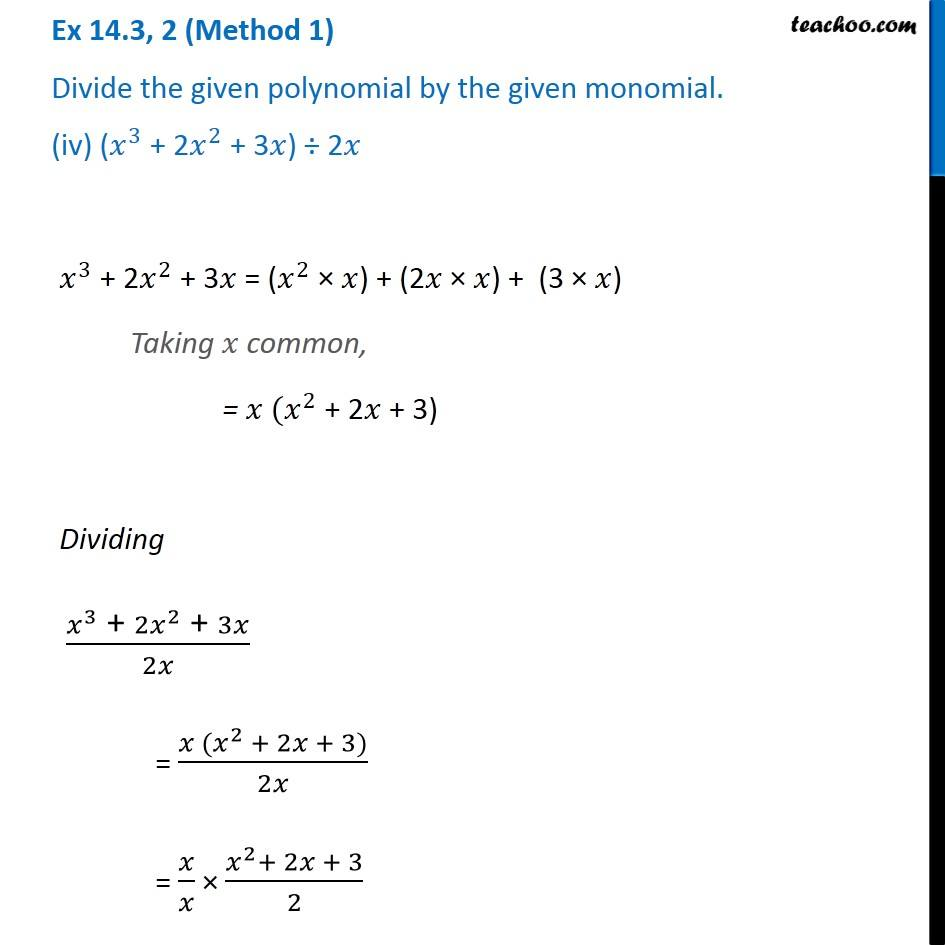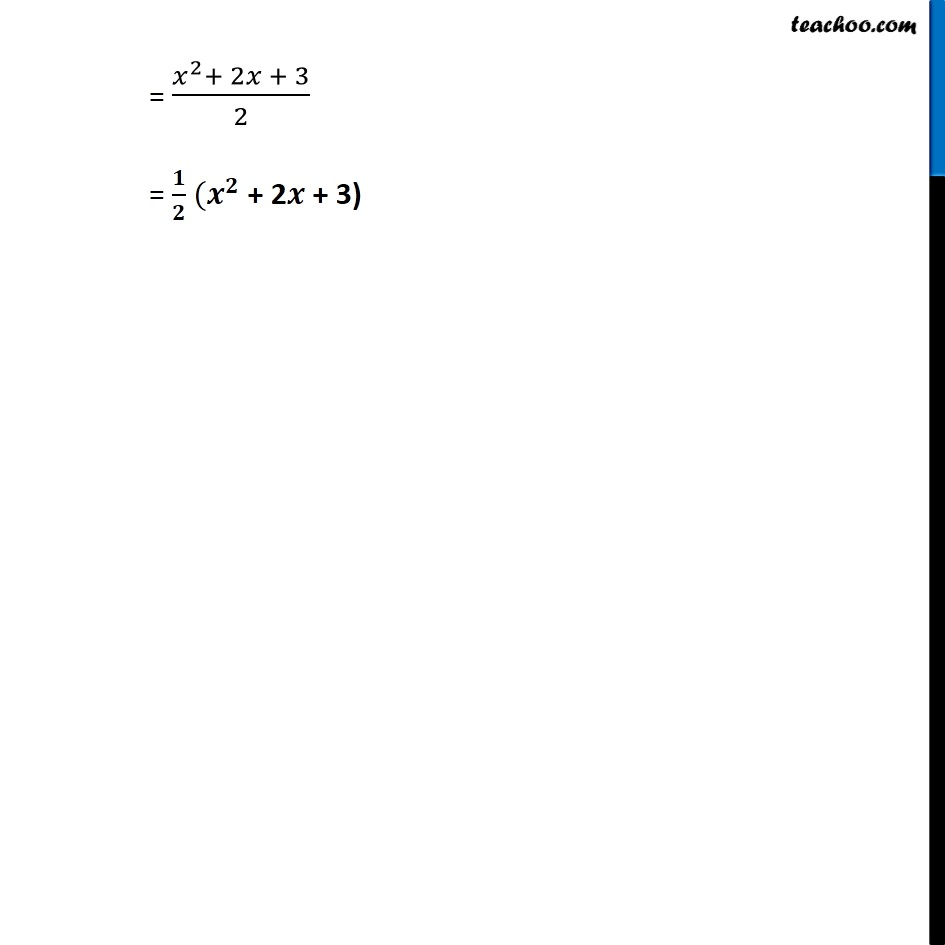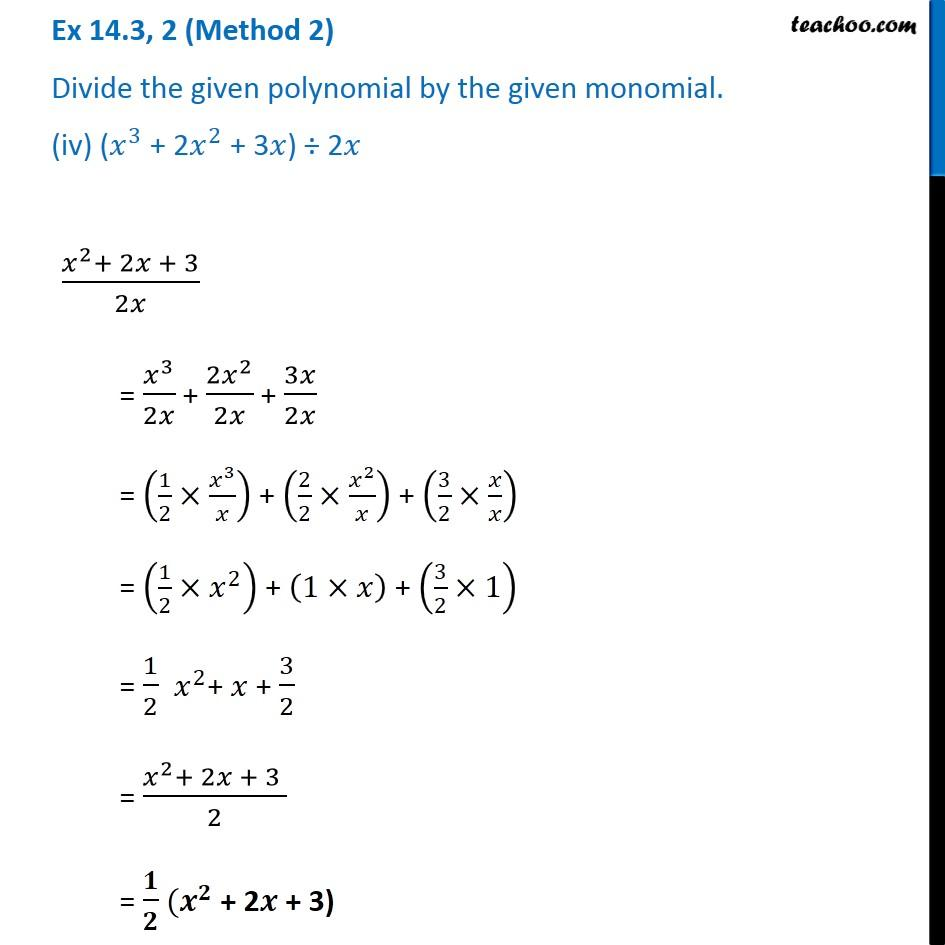Ex 14.3

Chapter 14 Class 8 Factorisation
Serial order wiseGet live Maths 1-on-1 Classs - Class 6 to 12

### Transcript

Ex 14.3, 2 (Method 1) Divide the given polynomial by the given monomial. (iv) (𝑥^3 + 2𝑥^2 + 3𝑥) ÷ 2𝑥 𝑥^3 + 2𝑥^2 + 3𝑥 = (𝑥^2 × 𝑥) + (2𝑥 × 𝑥) + (3 × 𝑥) Taking 𝑥 common, = 𝑥 (𝑥^2 + 2𝑥 + 3) Dividing (𝑥^3 " + " 2𝑥^2 " + " 3𝑥)/2𝑥 = (𝑥 (𝑥^2 + 2𝑥 + 3))/2𝑥 = 𝑥/𝑥 × (𝑥^2+ 2𝑥 + 3)/2 = (𝑥^2+ 2𝑥 + 3)/2 = 𝟏/𝟐 (𝒙^𝟐 + 2𝒙 + 3) Ex 14.3, 2 (Method 2) Divide the given polynomial by the given monomial. (iv) (𝑥^3 + 2𝑥^2 + 3𝑥) ÷ 2𝑥 (𝑥^2+ 2𝑥 + 3)/2𝑥 = 𝑥^3/2𝑥 + (2𝑥^2)/2𝑥 + 3𝑥/2𝑥 = (1/2×𝑥^3/𝑥) + (2/2×𝑥^2/𝑥) + (3/2×𝑥/𝑥) = (1/2×𝑥^2 ) + (1×𝑥) + (3/2×1) = 1/2 𝑥^2+ 𝑥 + 3/2 = (𝑥^2+ 2𝑥 + 3 )/2 = 𝟏/𝟐 (𝒙^𝟐 + 2𝒙 + 3)